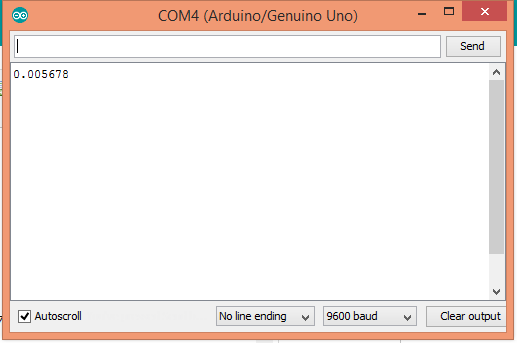# extracting last 4 digits after decimal comma

Hello everyone,

is there any function or a code that returnes the decimal digits of a float ?
for example: I have float A = 12.345678 but I'd like to get an int B = 5678 of that float.

P.S. I actually don't know the value of A, so multiplying A by 10e6 and then substracting 12340000 isn't possible. I just assigned a value to A here to make the problem clear, but it's for sure that the float value will have the same structure as A (two digits before the decimal point and 6 digits after it)

thanks a lot

I have float A = 12.345678 but I'd like to get an int B = 5678 of that float.

Tell us what you want to do.

If you have any float value, storing it in an int will give the integer portion.

``````float f = 12.345678;
int i = f; // i will be 12

float d = f - i; // d will be 0.345678
d *= 100; // d will be 34.5678

i = d; // i will be 34
d -= i; // d will now be 0.5678
d /= 100.0; // d will now be APPROXIMATELY 0.005678
``````

Or, it you want the value 5678 as an int, multiply by 10000 instead of dividing by 100.

Note that a float can not hold 8 digits of precision as your example alludes to.

WHY do you (think you) want to do this?

You need to understand that floats have only 6-7 decimal digits of precision but try this

``````void setup()
{
Serial.begin(115200);
Serial.println(getPart(12.345678));
Serial.println(getPart(12.987654));
Serial.println(getPart(12.321321));
}

void loop()
{
}

int getPart(float aFloat)
{
unsigned long aLong = aFloat * 1000000;
int anInt = aLong % 10000;
return anInt;
}
``````

I'm working with Latitudes and Longitudes and since processing floats takes more time that processing integers and due to the fact that the lats always have the same first 2 digits and the same first decimal digits (similiary lons), I thought that I could get rid of those digits and work with the last 4 or 5 decimal digits as integers

I'm working with Latitudes and Longitudes

That you get from? If they are coming from a GPS, they come as strings, so converting to floats and then to ints is going to loose precision.

The best approach would be not to convert them to floats in the first place.

I got the code from David Houlding's personal blog and both lats and lons are defined as floats in the code

MrMajd:
I got the code from David Houlding's personal blog and both lats and lons are defined as floats in the code

That's not an answer to anyone's question.

They're not floats in an NMEA sentence.

PaulS:
If you have any float value, storing it in an int will give the integer portion.

``````float f = 12.345678;
``````

int i = f; // i will be 12

float d = f - i; // d will be 0.345678
d *= 100; // d will be 34.5678

i = d; // i will be 34
d -= i; // d will now be 0.5678
d /= 100.0; // d will now be APPROXIMATELY 0.005678

Why are you saying 'APPROXIMATELY 0.005678'? It is exactly:0.005678.**BTW:**I have enjoyed a lot of the very rare instance of the teaching methodology of a Primary School Teacher. K+.PaulS:
That's not an answer to anyone's question.

you mentioned that lats and lons come as strings from GPS and converting them would loosen the accuracey.....so my reply to that: in the code from david, they are definded and returned as floats.

Why are you say 'APPROXIMATELY 0.005678'. It is exactly:0.005678.

Because it started off as a 32 bit floating point value.

Why are you say 'APPROXIMATELY 0.005678'. It is exactly:0.005678.

Nonsense. The initial value was NOT accurate to 8 digits, so the result, after several multiplications and divisions is not going to be more accurate.

MrMajd:
you mentioned that lats and lons come as strings from GPS and converting them would loosen the accuracey.....so my reply to that: in the code from david, they are definded and returned as floats.

That David's code allowed for inaccurate handling of lat/lon values does not mean that yours has to.

MrMajd:
you mentioned that lats and lons come as strings from GPS and converting them would loosen the accuracey.....so my reply to that: in the code from david, they are definded and returned as floats.

It pains me to say such an obvious thing, but "So, change the code from David (whoever he is)"

PaulS:
Nonsense.

Our Finance Minister, almost in all occasions, broadcasts the word Nonsense regardless of the contexts. People took a long time to discover that the word was not an insult to them; rather, the Minister was educated in the West where Nonsense is a vocabulary.

AWOL:
It pains me to say such an obvious thing, but "So, change the code from David (whoever he is)"

I'd like to, but I'm still a beginer in programming and the code he's a bit complicated for me, however it works

If you have the time, could you take a look at the code and help me get it exactly (for example, changing it so that lats and lons are strings and not floats) thanks